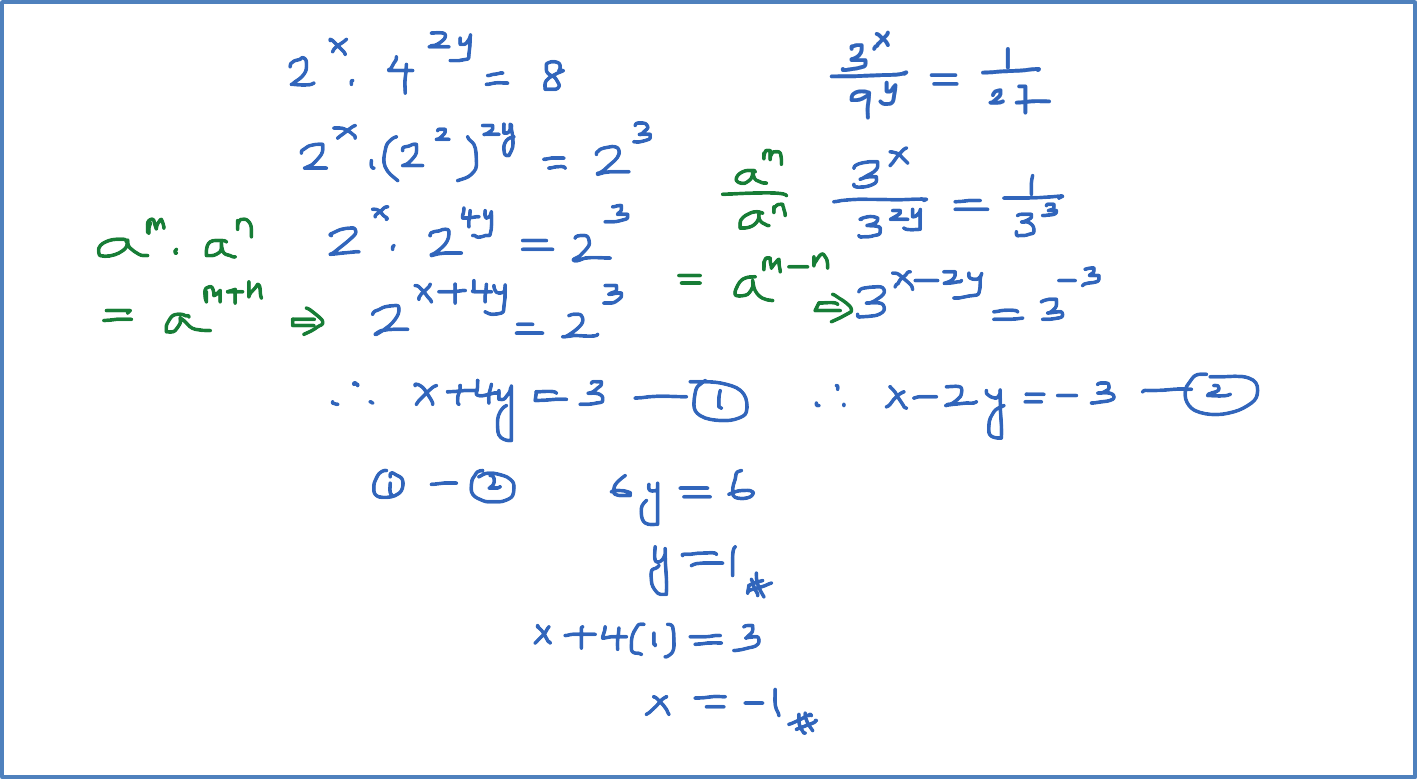# 5.3.3 Example 3 (Solving Index Equation Simultaneously)

Example 3 (Solving index equation simultaneously)
Solve the following simultaneous equations.

${2}^{x}{.4}^{2y}=8$

$\frac{{3}^{x}}{{9}^{y}}=\frac{1}{27}$### 1 thought on “5.3.3 Example 3 (Solving Index Equation Simultaneously)”

1.Please solve this simultaneously
2^2-x + 5^2-y = 7 and 5^y – 2^x+2 = -3